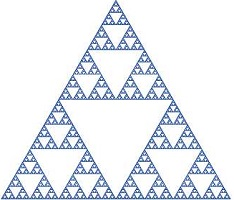# Java recursion exampleRecursion is when a function calls itself repeatedly. The classical example of recursion the factorial function.

``````5! = 5 x 4 x 3 x 2 x 1
n! = n * (n-1) * (n-2) * (n-3) * (n-4) * (n-5)
n! = n * (n-1)!
5! = 5 * 4!
5! = 5 * 4 * 3!
...``````

To create a recursive definition of this function, we need to define a recursive case and a base case.

``````recursive case: n! = n * (n - 1)! => fact(n) = n x fact(n -1) when n >= 1
base case: if n = 0, fact(n) = 1``````

The program

``````public class Factorial {
public static void main(String[] args) {
int n = 12;
System.out.println(n + "! = " + fact(n));
}

public static int fact(int n) {
if (n == 0) {
return 1;
} else {
return n * fact(n-1);
}
}
}``````

output

``12! = 479001600``